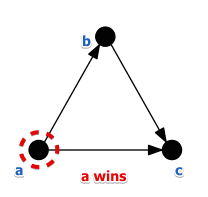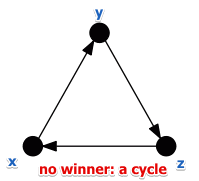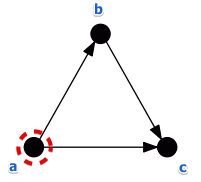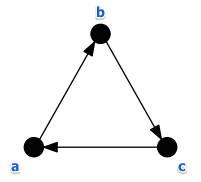# ML Wiki

## Condorcet's Rule

This a voting mechanism from Voting Theory.

The main idea:

• all candidates are compared in pairs
• prefer the candidate who are preferred by the majority in pair-wise comparison

### Fairness

We say that a voting system (procedure) is fair when

• if we choose a candidate $x$ then
• $x$ can beat every other candidate in a pair-wise election
• in this case $x$ is called the Condorcet winner

If $x$ wins the election but it loses in pairwise comparison

• then the Condorcet fairness criteria is not satisfied

Many other Voting Theory methods do not satisfy this criterion:

But the Condorcet Voting System (discussed below) does satisfy it.

### Condorcet Voting System

Idea:

• all candidates from the set $A$ are compared in pairs
• there are $N$ voters
• $n_{ij}$ is the number of voters that prefer $i$ to $j$ (i.e. they say $i > j$)
• $n_{ij} + n_{ji} = N$

$i$ is preferred globally to $j$ $\iff n_{ij} > \cfrac{N}{2}$

Preference Graph:

• we depict all preferences in a graph
• each candidate is a node
• an edge between two nodes $a$ and $b$ means "$a$ is preferred over $b$" ($a > b$)

We find the winner by looking for an node that has no incoming edges

• it means that this candidate is preferred to all other candidates

### Example

Individual rankings:

• $a > b > c$ - 11 voters
• $b > a > c$ - 8 voters
• $c > b > a$ - 2 voters

Pair-wise comparisons:

• $a \text{ vs } b$: $n_{ab} = 11, n_{ba} = 10 \Rightarrow a > b$
• $a \text{ vs } c$: $n_{ac} = 19, n_{ca} = 2 \Rightarrow a > c$
• $b \text{ vs } c$: $n_{bc} = 19, n_{cb} = 2 \Rightarrow b > c$

The Preference Graph for this example isThe Condorcet winner does not always exist - this is called the Condorcet Paradox.

• when there's a cycle in the Preference Graph - there is no winner

### Example

$A = \{x, y, z\}, N = 60$

Individual rankings:

• 23: $x > y > z$
• 17: $y > z > x$
• 2: $y > x > z$
• 10: $z > x > y$
• 8: $z > y > x$

The preference graph has a cycle:## Criteria

This rule satisfies:

Does not satisfy:

### Monotonicity

Suppose we have the following ranting:

• 1 vote: $a > b > c$
• 1 vote: $b > c > a$
• 1 vote: $a > c > b$

Let's build the preference graph:

• $a$ vs $b$: $n_{ab} = 2, n_{ba} = 1 \Rightarrow a > b$
• $a$ vs $c$: $n_{ac} = 2, n_{ca} = 1 \Rightarrow a > c$
• $b$ vs $c$: $n_{bc} = 2, n_{cb} = 1 \Rightarrow b > c$
•Suppose now $c$ improves his position:

• 1 vote: $a > b > c$
• 1 vote: $b > c > a$
• 1 vote: ${\color{blue}{c > a}} > b$

We have the following preference graph:

• $a$ vs $b$: $n_{ab} = 2, n_{ba} = 1 \Rightarrow a > b$
• $a$ vs $c$: $n_{ac} = 1, n_{ca} = 2 \Rightarrow {\color{blue}{c > a}}$
• $b$ vs $c$: $n_{bc} = 2, n_{cb} = 1 \Rightarrow b > c$
•• no one is the winner now - there is no solution

So by improving his position $c$ should stay in at least the same position

• but now he is not - everybody loses

### Separability

Say we have two regions $A$ and $B$, and $A \cup B = V$.

• suppose for both $A$ and $B$ we have same ranking: $a$ is preferred to $b$
• $n^A_{ab} > n^A_{ba}$ for $A$ and $n^B_{ab} > n^B_{ba}$ for $B$
• if we run the election in $V$ we will get:
• $n_{ab} = n^A_{ab} + n^B_{ab}$, $n_{ba} = n^A_{ba} + n^B_{ba}$
• $n^A_{ab} > n^A_{ba} \land n^B_{ab} > n^B_{ba} \Rightarrow n_{ab} > n_{ba}$
• so $a$ is preferred over $b$ in the whole region $V$ as well

Thus, Separability is respected.